# Short Answer Questions: Polynomials - 1 Notes | Study Mathematics (Maths) Class 10 - Class 10

## Class 10: Short Answer Questions: Polynomials - 1 Notes | Study Mathematics (Maths) Class 10 - Class 10

The document Short Answer Questions: Polynomials - 1 Notes | Study Mathematics (Maths) Class 10 - Class 10 is a part of the Class 10 Course Mathematics (Maths) Class 10.
All you need of Class 10 at this link: Class 10

Q 1. Find the sum and product of zeroes of 3x2 - 5x + 6.

Here, p (x) = 3x2 - 5x + 6
Comparing it with ax2 + bx + c, we have
a = 3, b = - 5, c = 6
∴ Sum of the zeroes =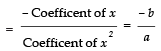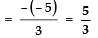and, Product of the zeroes =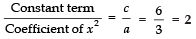Q 2. Find the sum and product of the zeroes of polynomial p (x) = 2x3 - 5x2 - 14x + 8.

Comparing p (x) = 2x3 - 5x2 - 14x + 8 with ax3 + bx2 + cx + d, we have
a = 2, b = –5,
c = - 14 and d = 8
∴ Sum of the zeroes =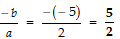Product of zeroes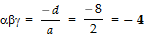Q 3. Find a Quadratic polynomial whose zeroes are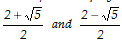.

Sum of zeroes (S)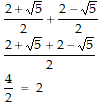Product of roots (P)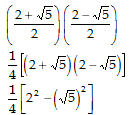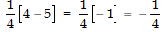= k(x2 - Sx + P) ; where k is any real number.

= k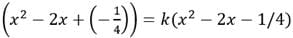Thus, the required polynomial is
= k (x2 - 2x - 1/4)

Q 4. If α and β are the zeroes of a Quadratic polynomial x2 + x - 2 then find the value of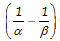.

Comparing x2 + x - 2 with ax2 + bx + c, we have:
a = 1, b = 1, c = - 2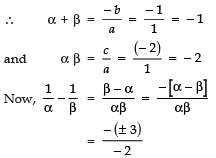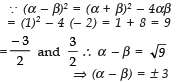Thus,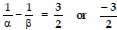Q 5. If a and b are the zeroes of x2 + px + q then find the value of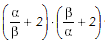.

Comparing x2 + px + q with ax2 + bx + c
a =1, b = p and c = q
∴ Sum of zeroes, a + b = - b/a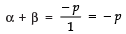and αβ = c/a
⇒ αβ = q/1 = q
Now,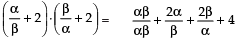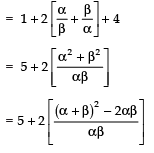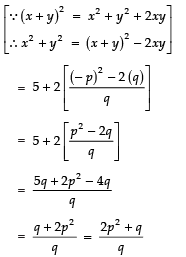Thus, the value of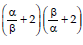is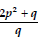Q 6. Find the zeroes of the quadratic polynomial 6x2 - 3 - 7x.

We have,
= 6x2 - 3 - 7x = 6x2 - 7x - 3
= 6x2 - 9x + 2x - 3
= 3x (2x - 3) + 1 (2x - 3)
= (3x + 1) (2x - 3)
For 6x2 - 3 - 7x to be equal to zero,
either (3x + 1) = 0 or (2x - 3) = 0
⇒ 3x = - 1 or 2x = 3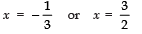Thus, the zeroes of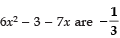and 3/2.

Q 7. Find the zeroes of 2x2 - 8x + 6.

We have,
2x2 - 8x + 6 = 2x2 - 6x - 2x + 6
= 2x (x - 3) - 2 (x - 3)
= (2x - 2) (x - 3)
= 2 (x - 1) (x - 3)
For 2x2 - 8x + 6 to be zero,
Either, x - 1 = 0 ⇒ x = 1
or x - 3 = 0 ⇒ x = 3
∴ The zeroes of 2x2 - 8x + 6 are 1 and 3.

Q 8. Find the zeroes of the quadratic polynomial 3x2 + 5x - 2.

We have,
p (x) = 3x2 + 5x - 2
= 3x2 + 6x - x - 2
= 3x (x + 2) - 1 (x + 2)
= (x + 2) (3x - 1)
For p (x) = 0, we get
Either x + 2 = 0 ⇒ x = - 2
or 3x - 1 = 0 ⇒ x = 1/3
Thus, the zeroes of 3x2 + 5x - 2 are - 2 and 1/3.

Q 9. If the zero of a polynomial p (x) = 3x2 - px + 2 and g (x) = 4x2 - q x - 10 is 2, then find the value of p and q.

∵ p (x) = 3x2 - px + 2
∴ p (2) = 3 (2)2 - p (2) + 2 = 0
[2 is a zero of p (x)]
or 12 - 2p + 2 or 14 - 2p = 0
or p = 7
Next g (x) = 4x2 - q x - 10
∴ g (2) = 4(2)2 - Q (2) - 10 = 0
[2 is a zero of g (x)]
or 4 × 4 - 2q - 10 = 0
or 16 - 2q - 10 = 0
or 6 - 2q = 0
⇒ q = 6/2 ⇒ q = 3
Thus, the required values are p = 7 and q = 3.

Q 10. Find the value of ‘k’ such that the quadratic polynomial 3x2 + 2kx + x - k - 5 has the sum of zeroes as half of their product.

Here, p (x) = 3x2 + 2kx + x - k - 5
= 3x2 + (2k + 1) x - (k + 5)
Comparing p (x) with ax2 + bx + c, we have:
a = 3, b = (2k + 1),
c = - (k + 5)
∴ Sum of the zeroes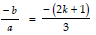Product of the zeroes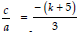According to the condition,
Sum of zeroes = 1/2 (product of roots)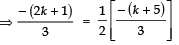⇒ - 2 (2k + 1) = - (k + 5)
⇒ 2 (2k + 1) = k + 5
⇒ 4k + 2 = k + 5
⇒ 4k - k = 5 - 2
⇒ 3k = 3
⇒ k = 3/3 = 1

Q 11. On dividing p (x) by a polynomial x - 1 - x2, the Quotient and remainder were (x - 2) and 3 respectively. Find p (x).

Here,dividend = p (x)
Divisor, g (x) = (x - 1 - x2)
Quotient, q(x) = (x - 2)
Remainder, r (x) = 3
∵ Dividend = [Divisor × Quotient] +Remainder
∴ p (x)= [g (x) × q(x)] + r (x)
= [(x - 1 - x2) (x - 2)] + 3
= [x2 - x - x3 - 2x + 2 + 2x2] + 3
= 3x2 - 3x - x3 + 2 + 3
= - x3 + 3x- 3x + 5

Q 12. Find the zeroes of the polynomial f (x) = 2 - x2.

We have f (x)= 2 - x2
= (√2 )- x2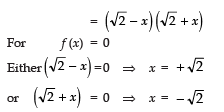Q 13. Find the cubic polynomial whose zeroes are 5, 3 and - 2.

∵ 5, 3 and - 2 are zeroes of p (x)
∴ (x - 5), (x - 3) and (x + 2) are the factors of p (x)
⇒ p (x) = k (x - 5) (x - 3) (x + 2)
= k (x2 - 8x + 15) (x + 2)
= k (x3 - 8x2 + 15x + 2x2 - 16x + 30
= k (x3 + [- 8 + 2] x2 + [15 - 16] x + 30)
= k (x- 6x2 - x + 30)
Thus, the required polynomial is k (x3 - 6x2 - x + 30).

Q 14. If α, β and γ be the zeroes of a polynomial p (x) such that (α + β +γ) = 3, (αβ + βγ + γα) = -10 and αβγ = - 24 then find p (x).

Here, α + β + γ = 3
αb + βγ + γα = - 10
αβγ = - 24
∵ A cubic polynomial having zeroes as α,β,γ is
p (x) = x3 - (a + b + γ) x+ (αβ + βγ + γα) x - (αβγ)
∴The required cubic polynomial is
= k {x3 - (3) x2 + (- 10) x - (- 24)}
= k(x- 3x2 - 10x + 24)

Note: If α, β and γ be the zeroes of a cubic polynomial p (x) then
p (x) = x3 - [Sum of the zeroes] x2 + [Product of the zeroes taken two at a time] x - [Product of zeroes]
i.e., p (x) = k {x3 - (α + β + γ) x2 + [αβ + βγ + γα] x - (αβγ).

Q 15. Find all the zeroes of the polynomial 4x4 - 20x3 + 23x2 + 5x - 6 if two of its zeroes are 2 and 3.

Here, p (x) =4x4 - 20x3 + 23x2 + 5x - 6
Since, 2 and 3 are the zeroes of p (x),
∴ (x - 2) and (x - 3) are the factors of p(x)
⇒ (x - 2) (x - 3) is a factor of p (x)
⇒ x- 5x + 6 is a factor of p (x)
Now, using the division algorithm for x2 - 5x + 6 and the given polynomial p (x), we
get: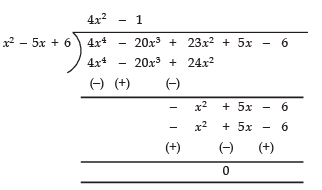∴ We get (x2 - 5x + 6) (4x2 - 1) = p (x)
⇒ (x - 3) (x - 2) [(2x)2 - (1)2] = p (x)
⇒ (x - 3) (x - 2) (2x - 1) (2x + 1) = p (x)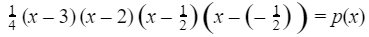Thus, all the zeroes of p (x) are: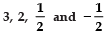Q 16. If 1 is a zero of x3 - 3x2 - x + 3 then find all other zeroes.

Here,p (x) = x3 - 3x2 - x + 3
∵ 1 is a zero of p (x)
∴ (x - 1) is a factor of p (x).
Now, dividing p (x) by (x - 1), we have: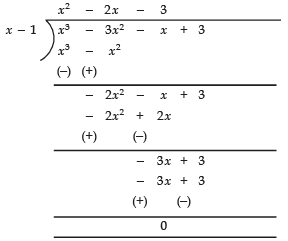∴ p (x) = (x - 1) (x2 - 2x - 3)
⇒ p (x) = (x - 1) [(x2 - 3x + x - 3)]
= (x - 1) [x (x - 3) + 1 (x - 3)]
= (x - 1) [(x - 3) (x + 1)]
i.e., (x - 1), (x - 3) and (x + 1) are the factors of p (x).
⇒ 1, 3, and - 1 are the zeroes of p (x).

Q 17. Find all the zeroes of 2x4 - 3x3 - 3x2 + 6x - 2, if two of its zeroes are 1 and 1/2.

Here, p(x) = 2x4 - 3x3 - 3x2 + 6x - 2
∵ 1 and are the zeroes of p (x)
∴ (x - 1) and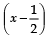are the factors of p (x)
⇒ (x - 1) (2x - 1) is a factor of p (x)
⇒ (2x2 - 3x + 1) is a factor of p (x).
Now, dividing p (x) by 2x2 - 3x + 1, we get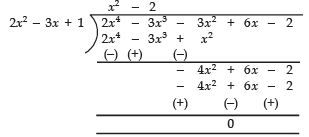∴ p (x) = (2x2 − 3x + 1) (x2 − 2)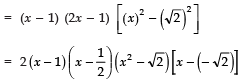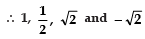are the zeroes of p (x).

Q 18. On dividing 4x3 - 8x2 + 8x + 1 by a polynomial g (x), the Quotient and remainder were (2x2 - 3x + 2) and (x + 3) respectively. Find g (x).

∵ Dividend = Divisor × Quotient + Remainder
i.e., p (x) = g (x) × Q (x) + r (x)
∴ g (x) =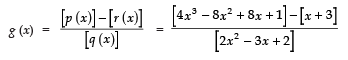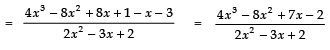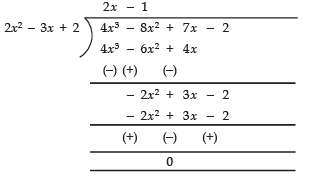Thus, the required polynomial g (x) = 2x - 1.

Q 19. If α and β are the zeroes of the quadratic polynomial p (x) = kx2 + 4x + 4 such that α2 + β2 = 24, find the value of k.

Here, p (x) = kx2 + 4x + 4.
Comparing it with ax2 + bx + c, we have:
a = k; b = 4; c = 4
∴ Sum of the zeroes = -b/a
⇒ α + β = -4/k
and Product of the zeroes = c/a
⇒ αβ = 4/k
∵ α2 + β= 24
∴ (α + β)2 - 2αβ = 24
[∵ (x + y)2 = x2 + y2 + 2xy ⇒ (x + y)2 - 2xy = x2 + y2]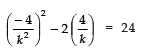⇒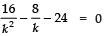⇒ 16 − 8k − 24k2 =0
⇒ 24k2 + 8k − 16 = 0
⇒ (3k − 2) (k + 1) = 0
⇒ 3k − 2= 0 or k + 1 = 0
⇒ k = 2/3 or k = -1

Q 20. Find the zeroes of the quadratic polynomial 6x2 - 3 - 7x and verify the relationship between the zeroes and the coefficients of the polynomial.

Here, p (x) = 6x2 - 3 - 7x = 6x2 - 7x - 3
= 6x- 9x + 2x - 3
= 3x (2x - 3) + 1 (2x - 3)
= (2x - 3) (3x + 1)
=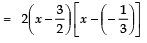∴ Zeroes of p (x) are 3/2 and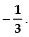To verify the relationship:
Sum of the zeroes =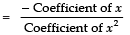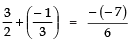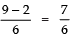⇒ 7/6 = 7/6
L.H.S = R.H.S ⇒ Relationship is verified.
Product of the zeroes =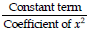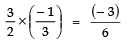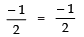i.e., L.H.S = R.H.S ⇒ Relationship is verified.

The document Short Answer Questions: Polynomials - 1 Notes | Study Mathematics (Maths) Class 10 - Class 10 is a part of the Class 10 Course Mathematics (Maths) Class 10.
All you need of Class 10 at this link: Class 10Use Code STAYHOME200 and get INR 200 additional OFF

## Mathematics (Maths) Class 10

58 videos|354 docs|138 tests

Track your progress, build streaks, highlight & save important lessons and more!

,

,

,

,

,

,

,

,

,

,

,

,

,

,

,

,

,

,

,

,

,

;